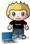# Convert to Decimal Degrees

posted in: Uncategorized | 0Useful little function and logic to convert to decimal degrees for use with Virtual Earth

``````
private double degreesMinutesSecondsToDecimal(string degminsec)
{
//Divide the number of seconds by 60, 28.79 ÷ 60 = 0.5166.
//Add the result to the number of minutes, 31 + .47983.
//Divide the result by 60, 31.47983 ÷ 60 = 0.52466383.
//Now add that to the number degrees, 48 + .52466383.
//The result is 48.52466383°

double seconds = Double.Parse(degminsec.Substring(degminsec.Length - 4, 4));
double minutes = Double.Parse(degminsec.Substring(degminsec.Length - 6, 2));
double degrees = Double.Parse(degminsec.Substring(0, degminsec.Length - 6));

if (degrees > 0)
{
return (((seconds / 100 / 60) + minutes) / 60) + degrees;
}
else
{
return degrees - (((seconds / 100 / 60) + minutes) / 60);
}
}
``````